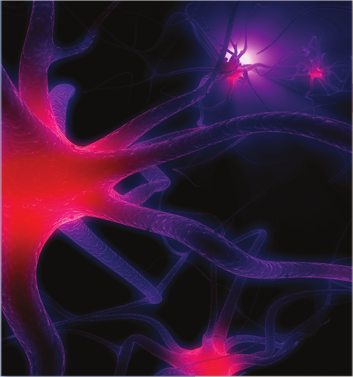We have used logarithms to solve exponential equations. In this chapter, we consider logarithmic functions as models in their own right. We also study another base for exponential and logarithmic functions, the natural base $$e\text{,}$$ which is the most useful base for many scientific applications.
 Time elapsed Percent remembered $$20$$ minutes $$58.2\%$$ $$1$$ hour $$44.2\%$$ $$9$$ hours $$35.8\%$$ $$1$$ day $$33.7\%$$ $$2$$ days $$27.8\%$$ $$6$$ days $$25.4\%$$ $$31$$ days $$21.1\%$$Ebbinghaus’s model uses a logarithmic function. The graph of the data, shown above with $$t$$ in minutes and $$y$$ in percents, is called the "forgetting curve." Ebbinghaus’s work, including his application of the scientific method to his research, provides part of the foundation of modern psychology.